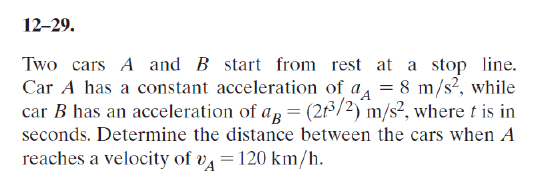# Question Solved1 AnswerPlease use the integration method. 12-29. Two cars A and B start from rest at a stop line. Car A has a constant acceleration of a a = 8 m/s2, while car B has an acceleration of ap = (26/2) m/s2, where t is in seconds. Determine the distance between the cars when A reaches a velocity of va = 120 km/h.E1IWNA The Asker · Mechanical EngineeringTranscribed Image Text: 12-29. Two cars A and B start from rest at a stop line. Car A has a constant acceleration of a a = 8 m/s2, while car B has an acceleration of ap = (26/2) m/s2, where t is in seconds. Determine the distance between the cars when A reaches a velocity of va = 120 km/h.
More
Transcribed Image Text: 12-29. Two cars A and B start from rest at a stop line. Car A has a constant acceleration of a a = 8 m/s2, while car B has an acceleration of ap = (26/2) m/s2, where t is in seconds. Determine the distance between the cars when A reaches a velocity of va = 120 km/h.